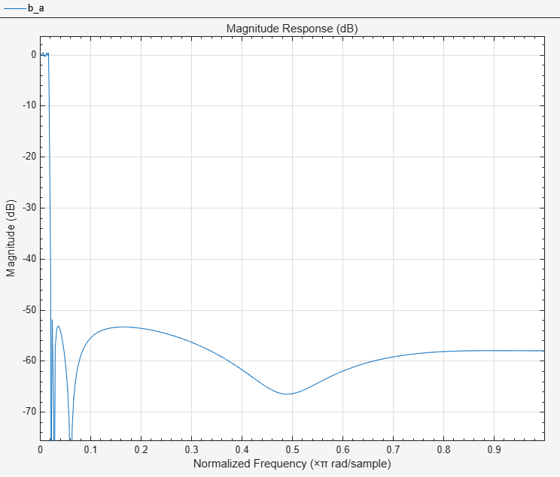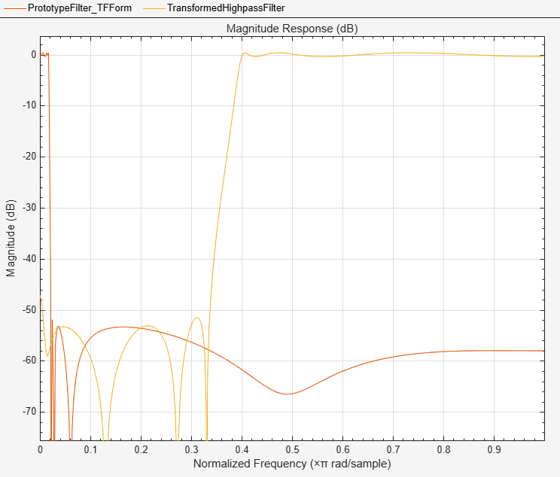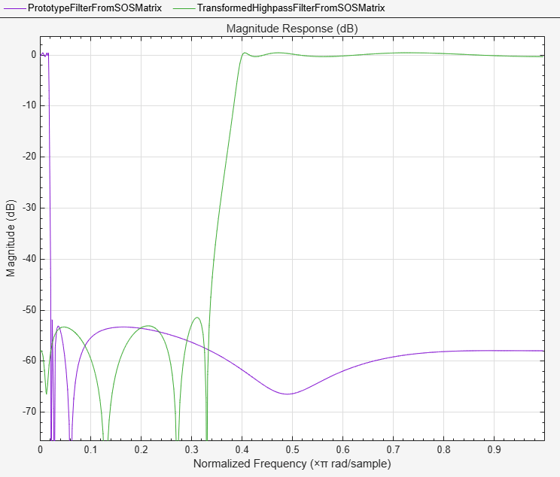# iirlp2hp

Transform lowpass IIR filter to highpass filter

## Syntax

``[num,den] = iirlp2hp(b,a,wo,wt)``
``````[num,den,allpassNum,allpassDen] = iirlp2hp(b,a,wo,wt)``````

## Description

example

````[num,den] = iirlp2hp(b,a,wo,wt)` transforms a lowpass IIR filter to a highpass IIR filter.The `iirlp2hp` function returns the numerator and denominator coefficients of the transformed highpass filter. The function accepts `wo`, frequency value to be transformed from the prototype filter, and `wt`, desired frequency in the transformed highpass filter, and applies the lowpass to highpass frequency transformation on the input prototype filter. The input prototype lowpass filter is specified with the numerator and denominator coefficients, `b` and `a` respectively. For more details on the transformation, see IIR Lowpass to Highpass Frequency Transformation. ```
``````[num,den,allpassNum,allpassDen] = iirlp2hp(b,a,wo,wt)``` additionally returns the numerator and the denominator coefficients of the mapping filter.```

## Examples

collapse all

Transform an IIR filter from lowpass to highpass by moving the magnitude response at one frequency in the source filter to a new location in the transformed filter.

Input Lowpass IIR Filter

Generate a least P-norm optimal IIR lowpass filter with varying attenuation levels in the stopband. Specify a numerator order of 10 and a denominator order of 6. The function returns the coefficients both in the vector form and in the second-order sections (SOS) form. The output argument `g` specifies the overall gain of the filter when expressed in the second-order sections form.

```[b,a,~,sos,g] = iirlpnorm(10,6,[0 0.0175 0.02 0.0215 0.025 1], ... [0 0.0175 0.02 0.0215 0.025 1],[1 1 0 0 0 0], ... [1 1 1 1 20 20]);```

Visualize the magnitude response of the filter.

`fvtool(b,a)`Transform Filter Using `iirlp2hp`

Transform the lowpass IIR filter using the `iirlp2hp` function. Specify the filter as a vector of numerator and denominator coefficients.

To generate a highpass filter whose passband flattens out at 0.4π rad/sample, select the frequency in the lowpass filter at 0.0175π, the frequency where the passband starts to roll off, and move it to the new location.

```wc = 0.0175; wd = 0.4; [num,den] = iirlp2hp(b,a,wc,wd);```

Compare the magnitude responses of the filters using FVTool. The transition band for the highpass filter is essentially the mirror image of the transition for the lowpass filter from 0.0175π to 0.025π, stretched out over a wider frequency range. In the passbands, the filter share common ripple characteristics and magnitude.

```hvft = fvtool(b,a,num,den); legend(hvft,"Prototype Filter (TF Form)",... "Transformed Highpass Filter")```Alternatively, you can also specify the input lowpass IIR filter as a matrix of coefficients. Pass the scaled second order section coefficient matrices as inputs. Apply the scaling factor `g` to the first section of the filter.

```sosg = sos; sosg(1,1:3) = g*sosg(1,1:3); [num2,den2] = iirlp2hp(sosg(:,1:3),sosg(:,4:6),wc,wd);```

Compare the magnitude response of the filters using FVTool.

```hvft = fvtool(sosg,[num2 den2]); legend(hvft,"Prototype Filter (SOS Form)",... "Transformed Highpass Filter")```## Input Arguments

collapse all

Numerator coefficients of the prototype lowpass IIR filter, specified as either:

• Row vector –– Specifies the values of [b0, b1, …, bn], given this transfer function form:

`$H\left(z\right)=\frac{B\left(z\right)}{A\left(z\right)}=\frac{{b}_{0}+{b}_{1}{z}^{-1}+\cdots +{b}_{n}{z}^{-n}}{{a}_{0}+{a}_{1}{z}^{-1}+\cdots +{a}_{n}{z}^{-n}},$`

where n is the order of the filter.

• Matrix –– Specifies the numerator coefficients in the form of an P-by-(Q+1) matrix, where P is the number of filter sections and Q is the order of each filter section. If Q = 2, the filter is a second-order section filter. For higher-order sections, make Q > 2.

`$b=\left[\begin{array}{ccccc}{b}_{01}& {b}_{11}& {b}_{21}& ...& {b}_{Q1}\\ {b}_{02}& {b}_{12}& {b}_{22}& ...& {b}_{Q2}\\ ⋮& ⋮& ⋮& \ddots & ⋮\\ {b}_{0P}& {b}_{1P}& {b}_{2P}& \cdots & {b}_{QP}\end{array}\right]$`

In the transfer function form, the numerator coefficient matrix bik of the IIR filter can be represented using the following equation:

`$H\left(z\right)=\prod _{k=1}^{P}{H}_{k}\left(z\right)=\prod _{k=1}^{P}\frac{{b}_{0k}+{b}_{1k}{z}^{-1}+{b}_{2k}{z}^{-2}+\cdots +{b}_{Qk}{z}^{-Q}}{{a}_{0k}+{a}_{1k}{z}^{-1}+{a}_{2k}{z}^{-2}+\cdots +{a}_{Qk}{z}^{-Q}},$`

where,

• a –– Denominator coefficients matrix. For more information on how to specify this matrix, see `a`.

• k –– Row index.

• i –– Column index.

When specified in the matrix form, b and a matrices must have the same number of rows (filter sections) Q.

Data Types: `single` | `double`
Complex Number Support: Yes

Denominator coefficients for a prototype lowpass IIR filter, specified as one of these options:

• Row vector –– Specifies the values of [a0, a1, …, an], given this transfer function form:

`$H\left(z\right)=\frac{B\left(z\right)}{A\left(z\right)}=\frac{{b}_{0}+{b}_{1}{z}^{-1}+\cdots +{b}_{n}{z}^{-n}}{{a}_{0}+{a}_{1}{z}^{-1}+\cdots +{a}_{n}{z}^{-n}},$`

where n is the order of the filter.

• Matrix –– Specifies the denominator coefficients in the form of an P-by-(Q+1) matrix, where P is the number of filter sections and Q is the order of each filter section. If Q = 2, the filter is a second-order section filter. For higher-order sections, make Q > 2.

`$a=\left[\begin{array}{ccccc}{a}_{01}& {a}_{11}& {a}_{21}& \cdots & {a}_{Q1}\\ {a}_{02}& {a}_{12}& {a}_{22}& \cdots & {a}_{Q2}\\ ⋮& ⋮& ⋮& \ddots & ⋮\\ {a}_{0P}& {a}_{1P}& {a}_{2P}& \cdots & {a}_{QP}\end{array}\right]$`

In the transfer function form, the denominator coefficient matrix aik of the IIR filter can be represented using the following equation:

`$H\left(z\right)=\prod _{k=1}^{P}{H}_{k}\left(z\right)=\prod _{k=1}^{P}\frac{{b}_{0k}+{b}_{1k}{z}^{-1}+{b}_{2k}{z}^{-2}+\cdots +{b}_{Qk}{z}^{-Q}}{{a}_{0k}+{a}_{1k}{z}^{-1}+{a}_{2k}{z}^{-2}+\cdots +{a}_{Qk}{z}^{-Q}},$`

where,

• b –– Numerator coefficients matrix. For more information on how to specify this matrix, see `b`.

• k –– Row index.

• i –– Column index.

When specified in the matrix form, a and b matrices must have the same number of rows (filter sections) P.

Data Types: `single` | `double`
Complex Number Support: Yes

Frequency value to transform from the prototype filter, specified as a real positive scalar in the range (`0`, `1`).

Data Types: `single` | `double`

Desired frequency location in the transformed highpass filter, specified as a real positive scalar in the range (`0`, `1`).

Data Types: `single` | `double`

## Output Arguments

collapse all

Numerator coefficients of the transformed highpass filter, returned as one of the following:

• Row vector of length n+1, where n is the order of the input filter. The `num` output is a row vector when the input coefficients `b` and `a` are row vectors.

• P-by-(Q+1) matrix, where P is the number of filter sections and Q is the order of each section of the transformed filter. The `num` output is a matrix when the input coefficients `b` and `a` are matrices.

Data Types: `single` | `double`
Complex Number Support: Yes

Denominator coefficients of the transformed highpass filter, returned as one of the following:

• Row vector of length n+1, where n is the order of the input filter. The `den` output is a row vector when the input coefficients `b` and `a` are row vectors.

• P-by-(Q+1) matrix, where P is the number of filter sections and Q is the order of each section of the transformed filter. The `den` output is a matrix when the input coefficients `b` and `a` are matrices.

Data Types: `single` | `double`

Numerator coefficients of the mapping filter, returned as a row vector.

Data Types: `single` | `double`

Denominator coefficients of the mapping filter, returned as a row vector.

Data Types: `single` | `double`

collapse all

### IIR Lowpass to Highpass Frequency Transformation

IIR lowpass to highpass frequency transformation takes a selected frequency from the lowpass filter, `wo`, and maps the corresponding magnitude response value onto the desired frequency location in the highpass filter, `wt`. Note that all frequencies are normalized between 0 and 1 and that the filter order does not change when you transform to a highpass filter.

When you select `wo` and designate `wt`, the transformation algorithm sets the magnitude response at the `wt` values of your bandstop filter to be the same as the magnitude response of your lowpass filter at `wo`. Filter performance between the values in `wt` is not specified, except that the stopband retains the ripple nature of your original lowpass filter and the magnitude response in the stopband is equal to the peak response of your lowpass filter. To accurately specify the filter magnitude response across the stopband of your bandpass filter, use a frequency value from within the stopband of your lowpass filter as `wo`. Then your bandstop filter response is the same magnitude and ripple as your lowpass filter stopband magnitude and ripple.

The transformation retains the shape of the original filter is what makes this function useful. If you have a lowpass filter whose characteristics, such as rolloff or passband ripple, particularly meet your needs, the transformation function lets you create a new filter with the same characteristic performance features, but in a highpass version without designing the highpass filter from the beginning.

In some cases transforming your filter may cause numerical problems, resulting in incorrect conversion to the highpass filter. Use `fvtool` to verify the response of your converted filter.

 Nowrouzian, B., and A.G. Constantinides. “Prototype Reference Transfer Function Parameters in the Discrete-Time Frequency Transformations.” In Proceedings of the 33rd Midwest Symposium on Circuits and Systems, 1078–82. Calgary, Alta., Canada: IEEE, 1991. https://doi.org/10.1109/MWSCAS.1990.140912.

 Nowrouzian, B., and L.T. Bruton. “Closed-Form Solutions for Discrete-Time Elliptic Transfer Functions.” In  Proceedings of the 35th Midwest Symposium on Circuits and Systems, 784–87. Washington, DC, USA: IEEE, 1992. https://doi.org/10.1109/MWSCAS.1992.271206.

 Constantinides, A.G.“Spectral transformations for digital filters.” Proceedings of the IEEE, vol. 117, no. 8: 1585-1590. August 1970.